Brooks (Base) Square (BS) 101

~ The Architecture of Space-Time (TAOST)

&

The Conspicuous Absence of Primes (TCAOP) ~

I.    TAOST / C. Geometrics – relationships / 1. Pythagorean

I. TAOST - the network

A. Geometrics – lines

B. Geometrics - shapes

C. Geometrics - relationships

1. Pythagorean <---

2. Parallelogram

3. Penta

II. TCAOP - everything minus the network

 TAOST: Rules 1-50 | Rules 51-80 | Rules 81-99 | Rules 100-107 | Rules 108-153 | TCAOP: Rule 154 | Rules 155-157 | Rules 158-159 | Rule 160 | Interconnectedness: Rules 161-175 | Appendix A: Rules 176-181 | Appendix B: Rules 182-200 |

I C. Geometrics - Relationships_1. Pythagorean

As we head deeper into the matrix, examining numerical relationships that include both lines and shapes that often move beyond the simple considerations we have touched on so far ... looking for those larger patterns. Hang on, for some of these new patterns are simply mind blowing in the majesty and beauty of their presence. What started as a simple table of relationships between the axial numbers and their square number counterparts depicting the Inverse Square Law (ISL) soon became much, much more.

How is it so? What is the mystery here in this magical mystery tour?

How does the Pythagorean Theorem (a2+b2=c2) appear?

Part 1-Pythagorean ((a2+b2=c2)

It does so in the form of triples ... Pythagorean triples ... of whole integer numbers. From Wikipedia (http://en.wikipedia.org/wiki/Pythagorean_theorem) we see a list of “Primitive Pythagorean triples up to 100.”

3,4,5 16,63,65

5,12,13 20,21,29

7,24,25 28,45,53

8,15,17 33,56,65

9,40,41 36,77,85

11,60,61 39,80,89

12,35,37 48,55,73

13,84,85 65,72,97

Yet it is multiples of the simplest ratio ... 3, 4, 5 ... that appear redundantly on the grid as an ever expanding pattern. Perhaps it is the very simplicity of the matrix that lends itself to the simplest of patterns.

It was the pattern itself that led down the path of parallelograms, in general, ultimately to that watershed of numerical relationships ... the penta- ... and the symmetry of numbers.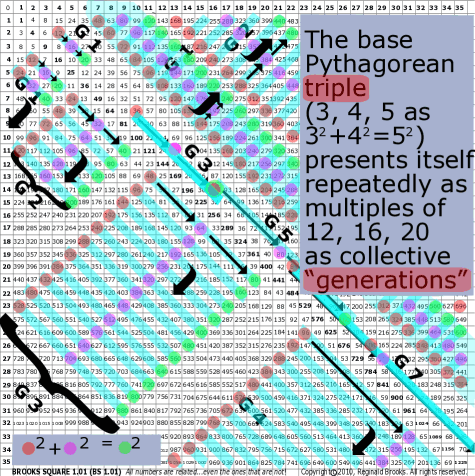~click to enlarge image 81 BS Rule 81: The base Pythagorean triple (3, 4, 5 as 32+42=52) presents itself repeatedly on Brooks (Base) Square as multiples of 12, 16, 20 as collective “generations.” These generations are oriented along both diagonals (like parallelograms) and distinguish themselves from each other by the number of diagonal steps between the constituent elements.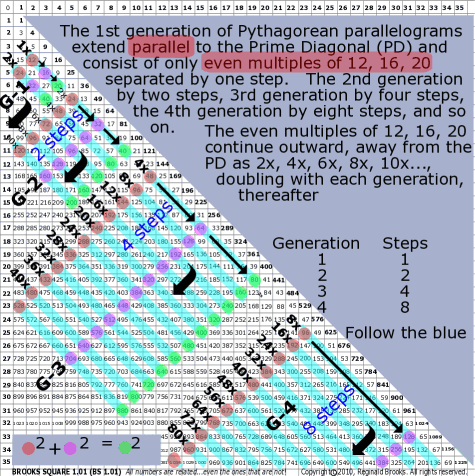~click to enlarge image
82

BS Rule 82: The 1st generation of Pythagorean parallelograms extend parallel to the Prime Diagonal (PD) and consist of only even multiples of 12, 16, 20 separated by one step. The 2nd generation by two steps, 3rd generation by four steps, the 4th generation by eight steps, and so on.

Note: The even multiples of 12, 16, 20 continue outward, away from the PD as 2x, 4x, 6x, 8x, 10x....

 Generation Steps 1 1 2 2 3 4 4 8

TOP ]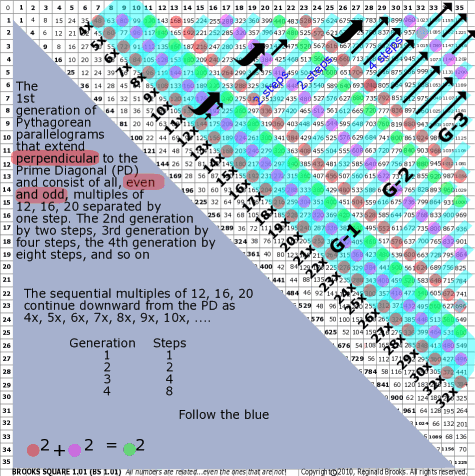~click to enlarge image
83

BS Rule 83: The 1st generation of Pythagorean parallelograms that extend perpendicular to the Prime Diagonal (PD) and consist of all, even and odd, multiples of 12, 16, 20 separated by one step. The 2nd generation by two steps, 3rd generation by four steps, the 4th generation by eight steps, and so on.

Note: The sequential multiples of 12, 16, 20 continue downward from the PD as 4x, 5x, 6x, 7x, 8x, 9x, 10x, ....

 Generation Steps 1 1 2 2 3 4 4 8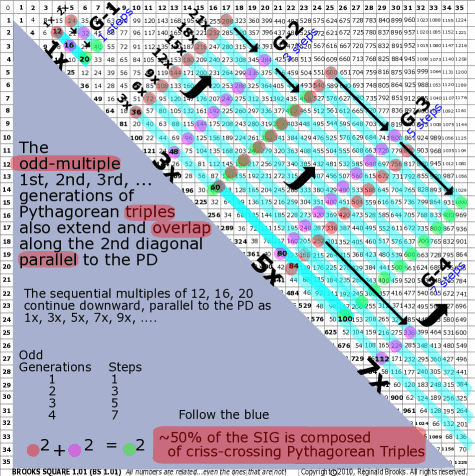~click to enlarge image
84

BS Rule 84: The odd-multiple 1st, 2nd, 3rd, ... generations of Pythagorean triples also extend and overlap along the 2nd diagonal parallel to the PD.

Note:

 Generation Steps 1 1 3 3 5 5 7 7 9 9

TOP ]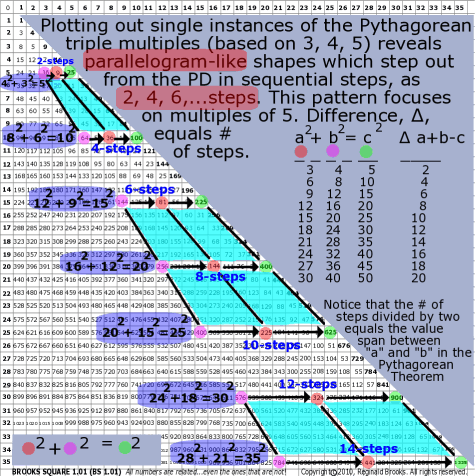~click to enlarge image
85

BS Rule 85: Plotting out single instances of the Pythagorean triple multiples (based on 3, 4, 5) reveals parallelogram-like shapes which step out from the PD in sequential steps, as 2, 4, 6,...steps.

Note: This pattern focuses on multiples of 5. Difference, ∆, steps.

 a2 + b2 = c2 ∆ a+b-c 3 4 5 2 6 8 10 4 9 12 15 6 12 16 20 8 15 20 25 10 18 24 30 12 21 28 35 14 24 32 40 16 27 36 45 18 30 40 50 20

TOP ]

 TAOST: Rules 1-50 | Rules 51-80 | Rules 81-99 | Rules 100-107 | Rules 108-153 | TCAOP: Rule 154 | Rules 155-157 | Rules 158-159 | Rule 160 | Interconnectedness: Rules 161-175 | Appendix A: Rules 176-181 | Appendix B: Rules 182-200 |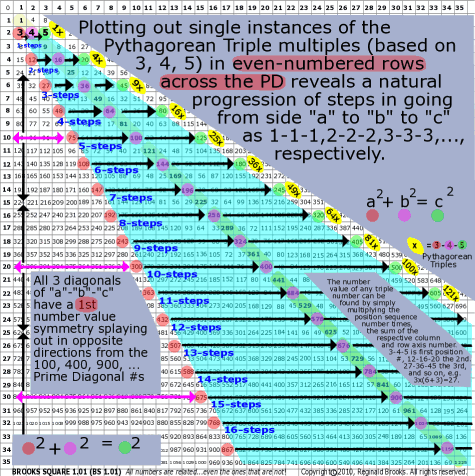~click to enlarge image
86

BS Rule 86: Plotting out single instances of the Pythagorean Triple multiples (based on 3, 4, 5) in even-numbered rows across the PD reveals a natural progression of steps in going from side "a" to "b" to "c" as 1-1-1,2-2-2,3-3-3,..., respectively.

Note: This pattern focuses on multiples of 2,3,4 and 5 in that each new Pythagorean Triple ... horizontally placed on a row, based on 3-4-5, that has side "a" in the lower SIG triangle, side "b" on the PD, and the hypotenuse, "c" in the upper SIG triangle... is located on the even numbered rows of the side axis. The first triple number ("a") is 3 and all subsequent "a's" are multiples of 3. The first "b" triple number is 4 and all subsequent "b's" on the PD are multiples of 4. The "c's" are all multiples of 5.

All 3 diagonals of "a"-"b"-"c" have a 1st number value symmetry splaying out in opposite directions from the 100, 400, 900, ... Prime Diagonal #s.

The number value of any triple number can be found by simply multiplying the position sequence number times, the sum of the respective column and row axis number. 3-4-5 is first position #, 12-16-20 the 2nd, 27-36-45 the 3rd, and so on, e.g. 3x(6+3)=27 and 3x(6+6)=36 and 3x(6+9)= 45 for the 3rd position Pythagorean Triple.

The triples follow a natural sequence of 1xP, 4xP, 9xP, 16xP, 25xP, 36xP,... where P= the original 3-4-5 Pythagorean Triple.

 a2 + b2 = c2 Steps 3 4 5 1 12 16 20 2 27 36 45 3 48 64 80 4 75 100 125 5 108 144 180 6 147 196 245 7 192 256 320 8 243 324 405 9 300 400 500 10

TOP ]

While I told myself I was not going to look for ... and describe ... any other simple number patterns before stopping and writing up what has been found thus far, I must digress ... briefly. This pattern below is so blatantly obvious, yet I didn’t see it before. It, along with a few other others ought to be in your, dear reader, toolbox before we really get into the best part of this tour.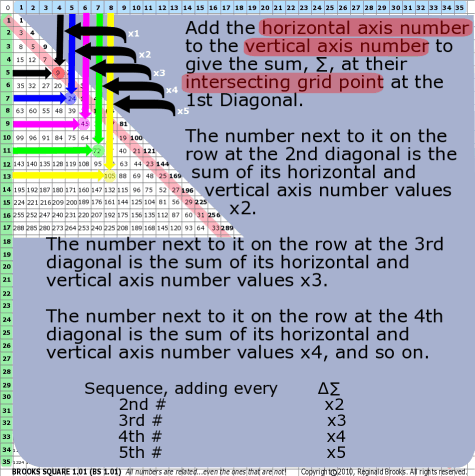~click to enlarge image
87

BS Rule 87: Add the horizontal axis number to the vertical axis number to give the sum, ∑, at their intersecting grid point at the 1st Diagonal.

Note: The number next to it on the row at the 2nd diagonal is the sum of its horizontal and vertical axis number values x2.

The number next to it on the row at the 3rd diagonal is the sum of its horizontal and vertical axis number values x3.

The number next to it on the row at the 4th diagonal is the sum of its horizontal and vertical axis number values x4, and so on.

Note:

 Sequence, adding every ∆∑ 2nd # x2 3rd # x3 4th # x4 5th # x5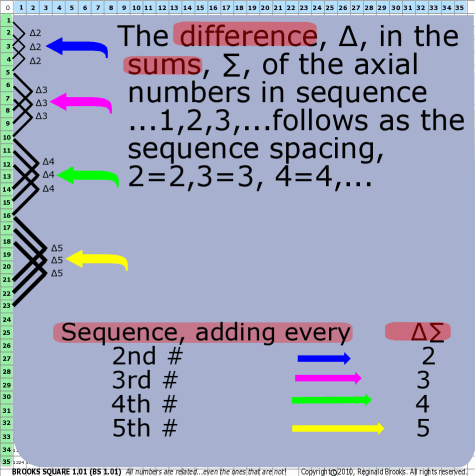~click to enlarge image
88

BS Rule 88: The difference, ∆, in the sums, ∑, of the axial numbers in sequence ...1,2,3,...follows as the sequence spacing, 2=2,3=3, 4=4,...

Note:

 Sequence, adding every ∆∑ 2nd # 2 3rd # 3 4th # 4 5th # 5

TOP ]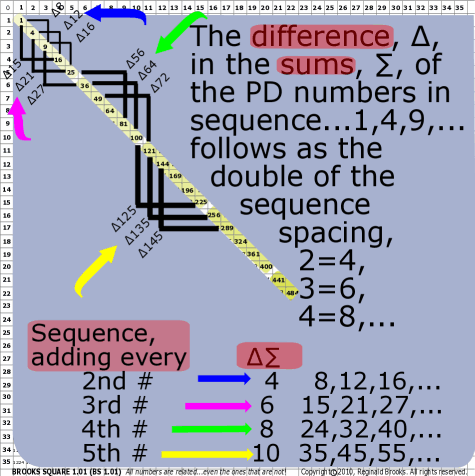~click to enlarge image
89

BS Rule 89: The difference, ∆, in the sums, ∑, of the PD numbers in sequence ...1,4,9,...follows as the double of the sequence spacing, 2=4,3=6, 4=8,...

Note:

 Sequence, adding every ∆∑ 2nd # 4 8,12,16,... 3rd # 6 15,21,27,... 4th # 8 24,32,40,... 5th # 10 35,45,55,...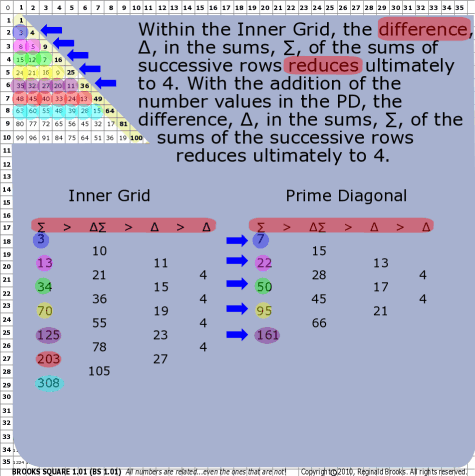~click to enlarge image
90

BS Rule 90: Within the Inner Grid, the difference, ∆, in the sums, ∑, of the sums of successive rows reduces ultimately to 4. With the addition of the number values in the PD, the difference, ∆, in the sums, ∑, of the sums of the successive rows reduces ultimately to 4.

Note: And includes every other odd # before reduction to 4.

 ∑ > ∆∑ > ∆ > ∆ 3 10 21 36 55 78 105 11 15 19 23 27 4 4 4 4 13 34 70 125 203 308

 ∑ > ∆∑ > ∆ > ∆ 7 15 28 45 66 13 17 21 4 4 22 50 95 161

TOP ]

 TAOST: Rules 1-50 | Rules 51-80 | Rules 81-99 | Rules 100-107 | Rules 108-153 | TCAOP: Rule 154 | Rules 155-157 | Rules 158-159 | Rule 160 | Interconnectedness: Rules 161-175 | Appendix A: Rules 176-181 | Appendix B: Rules 182-200 |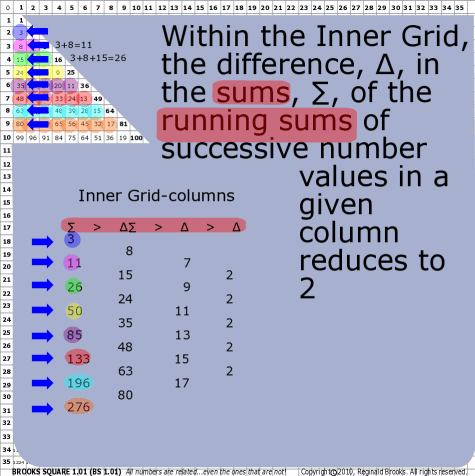~click to enlarge image
91

BS Rule 91: Within the Inner Grid, the difference, ∆, in the sums, ∑, of the running sums of successive number values in a given column reduces to 2.

Note: And includes every odd # before reduction to 2.

 ∑ > ∆∑ > ∆ > ∆ 3 8 15 24 35 48 63 7 9 11 13 15 2 2 2 2 11 26 50 85 133 196 276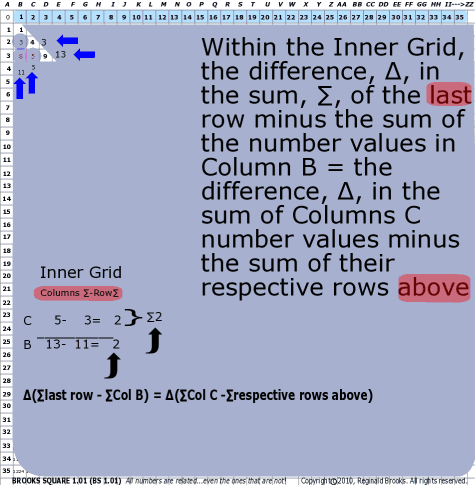~click to enlarge image 92 BS Rule 92: Within the Inner Grid, the difference, ∆, in the sum, ∑, of the last row minus the sum of the number values in Column B = the difference, ∆, in the sum of Column C number values minus the sum from the row above. Note: ∆(∑last row - ∑Col B) = ∆(∑Col C -∑row above)

TOP ]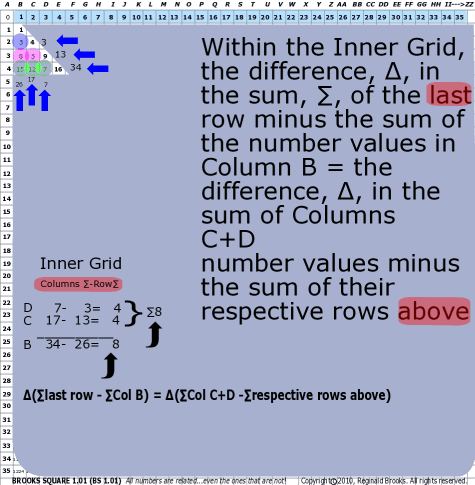~click to enlarge image 93 BS Rule 93: Within the Inner Grid, the difference, ∆, in the sum, ∑, of the last row minus the sum of the number values in Column B = the difference, ∆, in the sum of Columns C+D number values minus the sum from their respective rows above. Note: ∆(∑last row - ∑Col B) = ∆(∑Col C+D -∑respective rows above)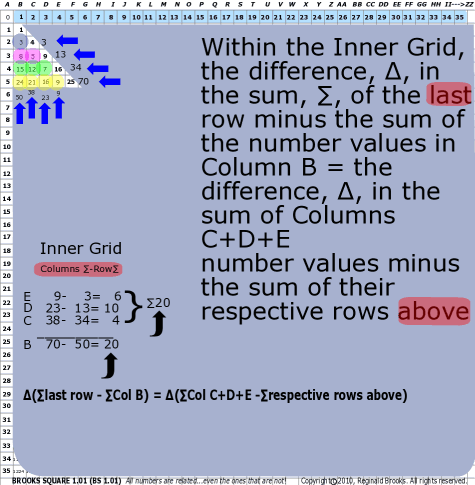~click to enlarge image 94 BS Rule 94:Within the Inner Grid, the difference, ∆, in the sum, ∑, of the last row minus the sum of the number values in Column B = the difference, ∆, in the sum of Columns C+D+E number values minus the sum of their respective rows above. Note: ∆(∑last row - ∑Col B) = ∆(∑Col C+D+E -∑respective rows above)

TOP ]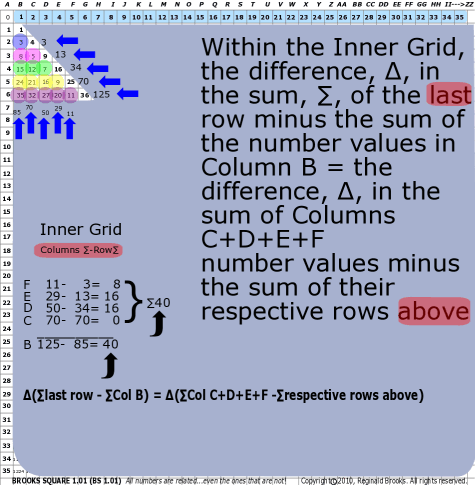~click to enlarge image 95 BS Rule 95: Within the Inner Grid, the difference, ∆, in the sum, ∑, of the last row minus the sum of the number values in Column B = the difference, ∆, in the sum of Columns C+D+E+F number values minus the sum of their respective rows above. Note: ∆(∑last row - ∑Col B) = ∆(∑Col C+D+E+F-∑respective rows above)

TOP ]

 TAOST: Rules 1-50 | Rules 51-80 | Rules 81-99 | Rules 100-107 | Rules 108-153 | TCAOP: Rule 154 | Rules 155-157 | Rules 158-159 | Rule 160 | Interconnectedness: Rules 161-175 | Appendix A: Rules 176-181 | Appendix B: Rules 182-200 |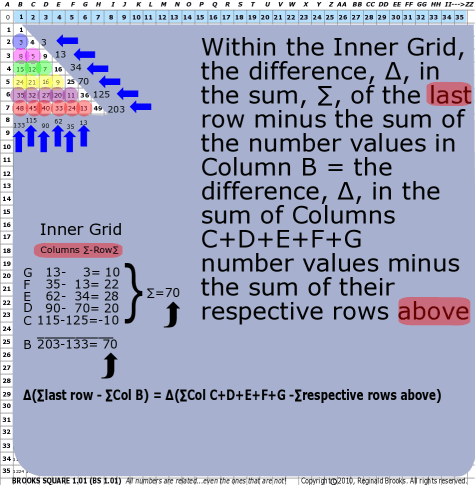~click to enlarge image 96 BS Rule 96: Within the Inner Grid, the difference, ∆, in the sum, ∑, of the last row minus the sum of the number values in Column B = the difference, ∆, in the sum of Columns C+D+E+F+G number values minus the sum of their respective rows above. Note: ∆(∑last row - ∑Col B) = ∆(∑Col C+D+E+F+G -∑respective rows above)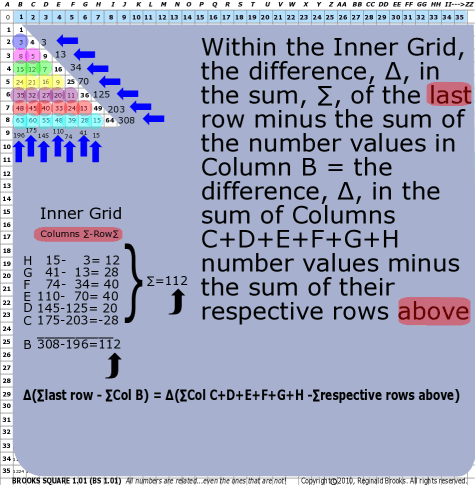~click to enlarge image 97 BS Rule 97: Within the Inner, the difference, ∆, in the sum, ∑, of the last row minus the sum of the number values in Column B = the difference, ∆, in the sum of Column C+D+E+F+G+H number values minus the sum of their respective rows above. Note: ∆(∑last row - ∑Col B) = ∆(∑Col C+D+E+F+G+H -∑respective rows above)

TOP ]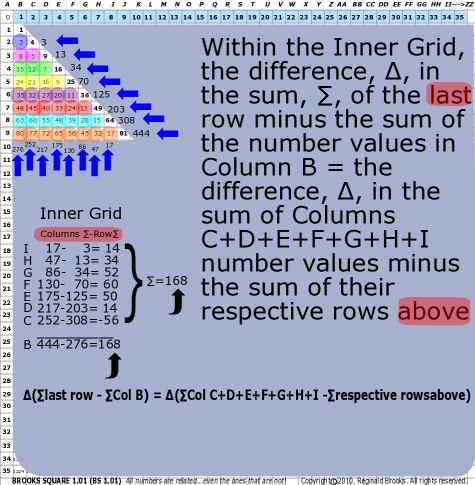~click to enlarge image 98 BS Rule 98: Within the Inner Grid, the difference, ∆, in the sum, ∑, of the last row minus the sum of the number values in Column B = the difference, ∆, in the sum of Columns C+D+E+F+G+H+I number values minus the sum of their respective rows above. Note: ∆(∑last row - ∑Col B) = ∆(∑Col C+D+E+F+G+H+I -∑respective rowsabove)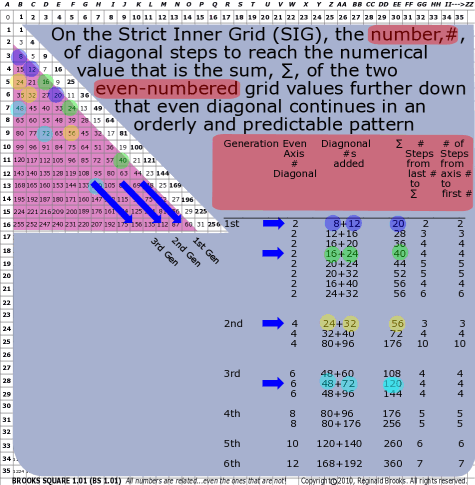~click to enlarge image
99

BS Rule 99: On the Strict Inner Grid (SIG), the number,#, of diagonal steps to reach the numerical value that is the sum, ∑, of the two even-numbered grid values further down that even diagonal continues in an orderly and predictable pattern.

Note:  Likewise, the # of steps down the diagonal to the first addition number grid value (starting with 2, it always being counted as two steps; starting with 4, it always being counted as 3 steps; starting with 6, it always being counted as 4 steps, and so on for each successive even diagonal) = the number of steps from the last addition number to the sum number of the two number values. Example: On the 2 diagonal: 28+32=60 and 60 is 7 steps down the diagonal from 32. It is also 7 steps from 2 ... the axial number topping the 8, 12, 16... diagonal, and remember it has a built-in count of 2, so 2 + 5=7 steps. If you go to the next even diagonal starting at axial number 4, its built-in step count is 3. Axial 6 has a built-in step count of 4, and so on. As the axial number grows by 2, the built-in step count grows by 1.

 Generation Even Axis # Diagonal Diagonal #s added ∑ # Steps from last # to  ∑ # of Steps from  axis #  to first # 1st 2 8+12 20 2 2 2 12+16 28 3 3 2 16+20 36 4 4 2 16+24 40 4 4 2 20+24 44 5 5 2 20+32 52 5 5 2 16+40 56 4 4 2 24+32 56 6 6 2nd 4 24+32 56 3 3 4 32+40 72 4 4 4 80+96 176 10 10 3rd 6 48+60 108 4 4 6 48+72 120 4 4 6 48+96 144 4 4 4th 8 80+96 176 5 5 8 80+176 256 5 5 5th 10 120+140 260 6 6 6th 12 168+192 360 7 7

TOP ]

 TAOST: Rules 1-50 | Rules 51-80 | Rules 81-99 | Rules 100-107 | Rules 108-153 | TCAOP: Rule 154 | Rules 155-157 | Rules 158-159 | Rule 160 | Interconnectedness: Rules 161-175 | Appendix A: Rules 176-181 | Appendix B: Rules 182-200 |

NEXT: On to I. TAOST>IC. Geometrics-relationships>IC2. Parallelograms - Brooks (Base) Square

Back to I. TAOST>IC. Geometrics-relationships- Brooks (Base) Square

Page 2a- PIN: Pattern in Number...from primes to DNA.

Page 2b- PIN: Butterfly Primes...let the beauty seep in..

Page 2c- PIN: Butterfly Prime Directive...metamorphosis.

Page 2d- PIN: Butterfly Prime Determinant Number Array (DNA) ~conspicuous abstinence~.

Page 3- GoDNA: the Geometry of DNA (axial view) revealed.

Page 4- SCoDNA: the Structure and Chemistry of DNA (axial view).

Page 5a- Dark-Dark-Light: Dark Matter = Dark Energy

Page 5b- The History of the Universe in Scalar Graphics

Page 5c- The History of the Universe_update: The Big Void

Page 6a- Geometry- Layout

Page 6b- Geometry- Space Or Time Area (SOTA)

Page 6c- Geometry- Space-Time Interactional Dimensions(STID)

Page 6d- Distillation of SI units into ST dimensions

Page 6e- Distillation of SI quantities into ST dimensions

Page 7- The LUFE Matrix Supplement: Examples and Proofs: Introduction-Layout & Rules

Page 7c- The LUFE Matrix Supplement: References

Page 8a- The LUFE Matrix: Infinite Dimensions

Page 9- The LUFE Matrix:E=mc2

Page 10- Quantum Gravity ...by the book

Page 11- Conservation of SpaceTime

Page 12- LUFE: The Layman's Unified Field Expose`

Page 13- GoMAS: The Geometry of Music, Art and Structure ...linking science, art and esthetics. Part I

Page 14- GoMAS: The Geometry of Music, Art and Structure ...linking science, art and esthetics. Part II

Page 15- Brooks (Base) Square (BS): The Architecture of Space-Time (TAOST) and The Conspicuous Absence of Primes (TCAOP) - a brief introduction to the series

Page 16- Brooks (Base) Square interactive (BBSi) matrix: Part I "BASICS"- a step by step, multi-media interactive

Page 17- The Architecture Of SpaceTime (TAOST) as defined by the Brooks (Base) Square matrix and the Inverse Square Law (ISL).